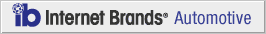# How Torque & Speed Work Together

January 27, 2012

If you have ever flicked through the pages of an automobile magazine and been confused by torque speed figures, fear no more. Below will give you all the basic information you require to understand these numbers. The level of torque force produced by a vehicle will grow with the size and power of the vehicle's engine and displays the level of turning force evident in the vehicle. Engine speed, the second of our two variables, is determined in RPM, with a higher number for more powerful vehicles. The two are reliant on each other and thus changing one will affect the other.

#### A Basic Equation

In order to better understand the relationship between torque and speed, you should learn the basic equation involving the two that allows you to work out the vehicle's horsepower:

(Torque x Engine Speed)/ 5,252 = Horsepower

This equation allows you to determine your vehicle's torque horsepower, which is the product of the unity of torque and speed. The calculation of the variable number (5,252 in this case) is easily obtained. Horsepower is measured as 550 foot-pounds/second. Next, use the radian of the wheel (half the circumference) divided by 60 i,n order to determine a dimensionless measurement that is measured in seconds. You multiply your RPM by your radian, producing 0.10472. Finally multiply this by your RPM to produce the dividing number at the end of the equation.

This equation highlights the relationship between the two variables and shows that the level of each torque and speed contributes wholly to the horsepower of your vehicle. Two invaluable forces reliant on physics dictate how your car produces its power.

COPYRIGHT 1999-2019 MH Sub I, LLC dba CarsDirect.com